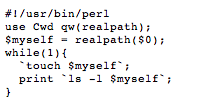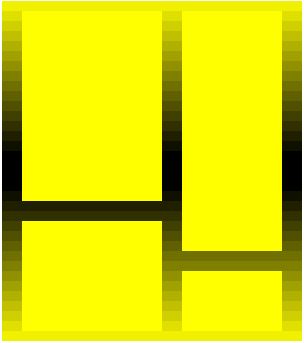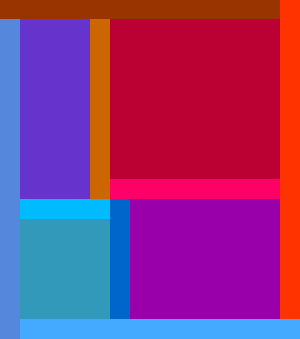(1)

(0)

## Seedbed (2009) - Pall Thayer

(1)More and more artists are producing art work through code and although very few of them see any reason to make the code central to an appreciation of the work, the very fact that code is being employed to produce art makes it relevant. There is meaning to be found within the codes regardless of what the artists themselves might say and the only way to emphasize this is to make the code central to an appreciation of the work. To put viewers in a position where they have to acknowledge the code and interact with the work at the code level before they are able to appreciate it at any other level.

-- FROM AN INTERVIEW WITH THE ARTIST ON CONT3XT.NET

MORE »

(2)

(0)

(0)

(0)

(1)

## Interview with JODI

(6)

fromjodi
toceci.moss
dateThu, Apr 2, 2009 at 3:07 AM
subjectdodo ¿ǝɯozıɥɹ ɹoɟ ʍǝıʌɹǝʇuı :ǝɹ

,ʍ, ,ʍ, ,ʍ, §=0 00==1 -17921790709oo<ʍ ɹɹ ʇǝʇǝ p n p nnpp,ʍ, ,ʍ, ,ʍ, §=0 00==1 -17921790709oo<ʍ ɹɹ ʇǝʇǝ p n p nnpp uoɔ 33b,ʍ, ,ʍ, ,ʍ, §=0 00==1 -17921790709oo<ʍ ɹɹ ʇǝʇǝ p n p nnpp uoɔ 33b,ʍ, ,ʍ, ,ʍ, §=0 00==1 -17921790709oo<ʍ ɹɹ ʇǝʇǝ p n p nnpp uoɔ 33b,ʍ, ,ʍ, ,ʍ, §=0 00==1 -17921790709oo<ʍ ɹɹ ʇǝʇǝ p n p nnpp uoɔ 33b,ʍ, ,ʍ, ,ʍ, §=0 00==1 -17921790709oo<ʍ ɹɹ ʇǝʇǝ p n p nnpp uoɔ 33b,ʍ, ,ʍ, ,ʍ, §=0 00==1 -17921790709oo<ʍ ɹɹ ʇǝʇǝ p n p nnpp uoɔ 33b,ʍ, ,ʍ, ,ʍ, §=0 00==1 -17921790709oo<ʍ ɹɹ ʇǝʇǝ p n p nnpp uoɔ 33b,ʍ, ,ʍ, ,ʍ, §=0 00==1 -17921790709oo<ʍ ɹɹ ʇǝʇǝ p n p nnpp uoɔ 33b,ʍ, ,ʍ, ,ʍ, §=0 00==1 -17921790709oo<ʍ ɹɹ ʇǝʇǝ p n p nnpp uoɔ 33b,ʍ, ,ʍ, ,ʍ, §=0 00==1 -17921790709oo<ʍ ɹɹ ʇǝʇǝ p n p nnpp uoɔ 33b,ʍ, ,ʍ, ,ʍ, §=0 00==1 -17921790709oo<ʍ ɹɹ ʇǝʇǝ p n p nnpp uoɔ 33b,ʍ, ,ʍ, ,ʍ, §=0 00==1 -17921790709oo<ʍ ɹɹ ʇǝʇǝ p n p nnpp uoɔ 33b,ʍ, ,ʍ, ,ʍ, §=0 00==1 -17921790709oo<ʍ ɹɹ ʇǝʇǝ p n p nnpp uoɔ 33b,ʍ, ,ʍ, ,ʍ, §=0 00==1 -17921790709oo<ʍ ɹɹ ʇǝʇǝ p n p nnpp uoɔ 33b,ʍ, ,ʍ, ,ʍ, §=0 00==1 -17921790709oo<ʍ ɹɹ ʇǝʇǝ p n p nnpp uoɔ 33b,ʍ, ,ʍ, ,ʍ, §=0 00==1 -17921790709oo<ʍ ɹɹ ʇǝʇǝ p n p nnpp uoɔ 33b,ʍ, ,ʍ, ,ʍ, §=0 00==1 -17921790709oo<ʍ ɹɹ ʇǝʇǝ p n p nnpp ...

MORE »

## Look, See (2008-Ongoing) - Chris Ashley

(13)20090330, 20090330, HTML, 340 x 300 pixels20090328, 20090328, HTML, 340 x 300 pixels

It is my intention to continue making an HTML drawing for every day in 2008 and beyond.